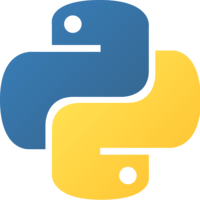Unlock This Lesson
1×
Become a member
to unlock all featuresLevel Up!

Access all courses & lessons on egghead today and lock-in your price for life.

Autoplay

# Find Clusters of Data with K-means Clustering in Python and Scikit-learnScikit-Learnpython

We’ll return to the iris dataset to see how to use k-means clustering, an unsupervised learning algorithm, to create categories for data that doesn't have labels. We'll also visualize these clusters using matplotlib.

More on K-means can be found at Scikit-learn.### Code### You must be a Member to view code

Access all courses and lessons, track your progress, gain confidence and expertise.

Become a Member
and unlock code for this lesson### Discuss### Transcript

Instructor: From scikit-learn, we'll import datasets. From sklearn.cluster, we'll import k-means. We'll import matplotlib.pyplot as plt. We'll be working with the iris dataset, which is datasets.load_iris. Let's print out our feature names.

We can see we have four features here. K-means is an unsupervised algorithm, which means that it's used on data that doesn't have labels. Even though this dataset has target labels, we're going to be ignoring it for the purpose of teaching k-means.

We'll assign our X to be iris.data. For simplicity's sake, we're just going to take two features to work with. We'll take the middle two features. From here, we can say model equals k-means.

K-means is a clustering algorithm, which means that we give it a number of clusters, and it figures out how to divide the data into that many clusters. It does this by creating centroids which are set to the mean of the cluster that it's defining. Let's see how that works.

If we say n_clusters, our number of clusters, equals 5, and we'll also pass in a random state, which will be 0and then we say model.fit and pass it our X data, then if we print model.labels -- this is supposed to be n_clusters -- then we can see the k-means model has taken our X data and assigned a label from 0to 4 for each data point.

Even though k-means is not a classification tool, this is the same as saying model.predict(X). We can also print model.clustercenters. These are the centroids of each delineated cluster. Let's visualize these.

We'll say plt.scatter. First, let's plot our X data, so the first variable and the second variable. We'll say the color is blue. We'll do an X label and a y label. Then we'll say plt.show. This has an underscore at the end of it. This is how two features of the data look plotted. If we want to add our centroids, we can say plt.scatter(centroids), first variable, and second variable.

We can say marker equals and then whatever shape we want it to look like, size equals 170, zorder equals 10 so that it will come to the top, and then color equals magenta. Let's also change our data color to be the model.labels. This will be the color of the cluster that k-means has put the data point into.

Here we can see the five groups that k-means has clustered this dataset into. If you thought this looked good, you could investigate further, and look for patterns and similar variables, and try to find connections that you might not have otherwise, but looking at this, it looks a little arbitrary.

It's also worth playing around with the number of clusters. We can see that this looks a little more intuitively separated. K-means is a good tool for exploring your data and for creating classes and labels if your dataset doesn't have them.